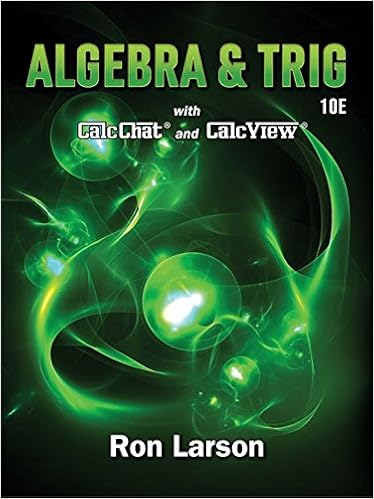# LinesAnglesPlanesTriangles.ppt - Points Points do not have...

• 61

This preview shows page 1 - 5 out of 61 pages.

##### We have textbook solutions for you!
The document you are viewing contains questions related to this textbook.The document you are viewing contains questions related to this textbook.
Chapter 6 / Exercise 01
Algebra & Trigonometry
LarsonExpert Verified
Lesson 1-1 Point, Line, Plane 1 Points Points do not have actual size. How to Sketch: Using dots How to label: Use capital letters Never name two points with the same letter (in the same sketch). A B A C
##### We have textbook solutions for you!
The document you are viewing contains questions related to this textbook.The document you are viewing contains questions related to this textbook.
Chapter 6 / Exercise 01
Algebra & Trigonometry
LarsonExpert Verified
Lesson 1-1 Point, Line, Plane 2 Lines Lines extend indefinitely and have no thickness or width. How to sketch : using arrows at both ends. How to name: 2 ways (1) small script letter – line n (2) any two points on the line - Never name a line using three points - , , , , , AB BC AC BA CA CB suu r suu r suur suu r suu r suu r n A B C ABC suuuu r
Lesson 1-1 Point, Line, Plane 3 Collinear Points Collinear points are points that lie on the same line. (The line does not have to be visible.) A point lies on the line if the coordinates of the point satisfy the equation of the line. Ex: To find if A (1, 0) is collinear with the points on the line y = -3x + 3. Substitute x = 1 and y = 0 in the equation. 0 = -3 (1) + 3 0 = -3 + 3 0 = 0 The point A satisfies the equation, therefore the point is collinear with the points on the line. A B C A B C Collinear Non collinear
Postulate 1-5 Ruler Postulate The distance between any two points is the absolute value of the difference of the corresponding numbers (on a number line or ruler) Congruent Segments : two segments with the same length
•••